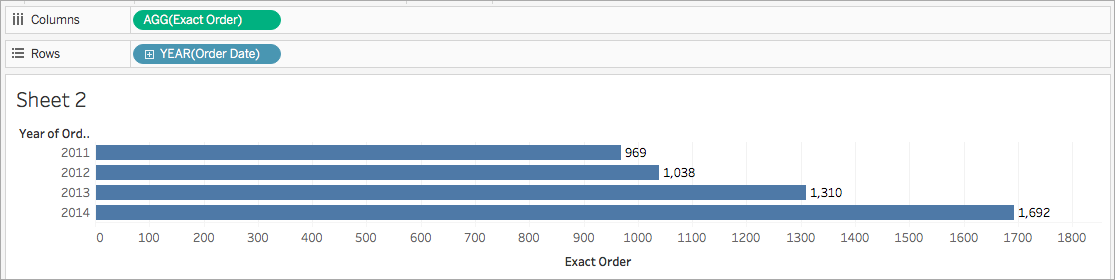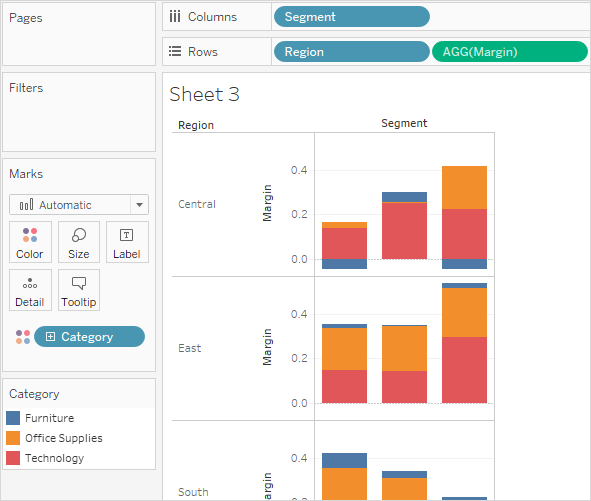## 為何使用彙總函式

`COUNTD(Order ID)`## Tableau 中的可用彙總函式

#### 定義

ATTR

`ATTR(expression)`

AVG

`AVG(expression)`

`COLLECT (spatial)`

`COLLECT ([Geometry])`

CORR

`CORR(expression 1, expression2)`

CORR 可用於以下資料來源：

• Tableau 資料擷取（您可以從任何資料來源中建立擷取）
• Cloudera Hive
• EXASolution
• Firebird（版本 3.0 及更高版本）
• IBM PDA (Netezza)
• Oracle
• PostgreSQL
• Presto
• SybaseIQ
• Vertica

`{CORR(Sales, Profit)}`

COUNT

`COUNT(expression)`

COUNTD

`COUNTD(expression)`

COVAR

`COVAR(expression 1, expression2)`

COVAR 可用於以下資料來源：

• Tableau 資料擷取（您可以從任何資料來源中建立擷取）
• Cloudera Hive
• EXASolution
• Firebird（版本 3.0 及更高版本）
• IBM PDA (Netezza)
• Oracle
• PostgreSQL
• Presto
• SybaseIQ
• Vertica

`COVAR([Sales], [Profit])`

COVARP

`COVARP(expression 1, expression2)`

COVARP 可用於以下資料來源：

• Tableau 資料擷取（您可以從任何資料來源中建立擷取）
• Cloudera Hive
• EXASolution
• Firebird（版本 3.0 及更高版本）
• IBM PDA (Netezza)
• Oracle
• PostgreSQL
• Presto
• SybaseIQ
• Vertica

`COVARP([Sales], [Profit])`

MAX

`MAX(expression)`

MEDIAN

`MEDIAN(expression)`

• Access
• Amazon Redshift
• HP Vertica
• IBM DB2
• IBM PDA (Netezza)
• Microsoft SQL Server
• MySQL
• SAP HANA

MIN

`MIN(expression)`

PERCENTILE

`PERCENTILE(expression, number)`

• 非舊版 Microsoft Excel 和文字檔連線。

• 擷取和純擷取資料來源類型（例如 Google Analytics (分析)、OData 或 Salesforce）。

• Sybase IQ 15.1 及更高版本的資料來源。

• Oracle 10 及更高版本的資料來源。

• Cloudera Hive 和 Hortonworks Hadoop Hive 資料來源。

• EXASolution 4.2 及更高版本的資料來源。

STDEV

`STDEV(expression)`

STDEVP

`STDEVP(expression)`

SUM

`SUM(expression)`

VAR

`VAR(expression)`

VARP

`VARP(expression)`

## 建立彙總計算

1. 在 Tableau Desktop 中，連線到 Tableau 附帶的 [Sample - Superstore] 已儲存資料來源。

2. 巡覽到工作表，並選取 [分析] > [建立計算欄位]

3. 在開啟的計算編輯器中，執行以下操作：

• 將計算欄位命名為 [Margin] （利潤）

• 輸入以下公式：

```IIF(SUM([Sales]) !=0, SUM([Profit])/SUM([Sales]), 0)```

附註：您可以使用函數引用來尋找彙總函式和其他函數（如此範例中的邏輯 IIF 函數），並將其新增到計算公式。有關詳情，請參閱在計算編輯器中使用函數引用

• 完成後，按一下 [確定]

新的彙總計算將出現在 [資料] 窗格中的 [度量] 下。就像其他欄位一樣，您可以在一個或多個視覺效果中使用該欄位。

附註：彙總計算始終為度量。

當將 [Margin] （利潤）放在工作表中的架或卡上時，它的名稱將變更為 [AGG(Margin)] ，表示它是彙總計算，並且無法進一步彙總。## 彙總計算的規則

• 任何彙總計算中不得同時包括彙總值和分解值。例如，SUM(Price)*[Items] 不是有效的運算式，因為 SUM(Price) 已彙總，而 Items 則沒有。不過，SUM(Price*Items) 和 SUM(Price)*SUM(Items) 均有效。

• 運算式中的常量可根據情況充當彙總值或分解值。例如：SUM(Price*7) 和 SUM(Price)*7 均為有效的運算式。

• 所有函數都可用彙總值進行計算。但是，任何給定函數的參數必須或者全部彙總，或者全部分解。例如：MAX(SUM(Sales),Profit) 不是有效的運算式，因為 Sales 已彙總，而 Profit 則沒有。不過，MAX(SUM(Sales),SUM(Profit)) 為有效的運算式。

• 彙總計算的結果始終為度量。

• 與預定義彙總一樣，彙總計算可正確地進行總計計算。有關詳情，請參閱 [總計] 。

## 另請參閱

Tableau 中的資料彙總(連結在新視窗開啟)

Tableau 中的函數

Tableau 函數（按類別）(連結在新視窗開啟)

Tableau 函數（按字母順序）